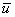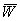# Test Case

## Brief description of the study test case

The geometry of the conical diffuser with key dimensions identified is illustrated in Figure 3 where a section is taken along the diffuser centre line.

Figure 3 Section Through the Centreline of the Conical Diffuser

As can be seen from Figure 3, the inlet section to the diffuser is 0.26m in diameter and this section extends for 0.025m upstream of the entry to the diffuser which has a 20° included angle and an area ratio of 2.86. The diffuser discharges to atmosphere and the downstream diameter of the diffuser is 0.44m.

The fluid simulated was air and the kinematic viscosity, v, specified was 1.5e-5m2/s. The average axial velocity at the inlet was 11.6m/s (U0). The corresponding Reynolds number (U0D/v) was 202,000. The tangential velocity distribution at inlet approximated that of a forced vortex, with a swirl number of order 0.35 based on the ratio of tangential momentum to axial momentum. This corresponds to a rather moderate degree of swirl.

The assessment quantities by which the success or failure of the CFD calculations were judged include velocity, Reynolds stress, turbulent kinetic energy, wall shear stress and static pressure coefficient measurements.

## Test Case Experiments

Details of the test case experiments can be found on the ERCOFTAC database and hence only an overview summary is provided here. Figure 4 illustrates the experimental set-up.

Figure 4 Experimental Set-up 

A rotating swirl generator with diameter 0.26m and including a honeycomb screen at its inlet to generate the swirl is located upstream of the conical diffuser. The dimensions and specifications of the conical diffuser are the same as those used as the test case CFD study (section 3) and shown in Figure 3.

Hot wire velocity measurements were carried out using a single wire probe for the mean quantities and an X-wire probe for the turbulence quantities. Static pressure measurements were recorded using wall taps. Measurements were recorded at seven stations along the diffuser. With the diffuser inlet defined as x=0mm, the stations were located at x=25mm, 50mm, 100mm, 175mm, 250mm, 330mm and 405mm downstream of the inlet. Specific quantities included:

Velocity measurements: Profiles perpendicular to the diffuser wall of first order moments from wall to center line,/U0,/U0, second order moments, Reynolds stresses, turbulent kinetic energy (deduced), wall stream line angle and distribution of wall shear stress

Pressure measurements: Distribution of the static pressure coefficient

The following measurement errors were identified:

δ (Reynolds stresses) 10%

δ (positions) ± 0.01mm

The flow boundary conditions at the inlet are specified explicitly and in a format to facilitate CFD calculations.

## CFD Methods

Unless stated otherwise, the description that follows is taken from the work of Armfield et al .

Geometry

The geometry of the CFD model was 2D axi-symmetric and was based on the geometry of Clausen et al  and shown in Figure 3.

Computational Grid

In the work reported by Armfield et al , results were obtained from a 40 x 40 quadrilateral element mesh. Grid independence was checked using 20 x 24 to 40 x 60 grids and the 40 x 40 grid results showed numerical errors in the mean flow quantities of less than 2% and in the near wall peaks of turbulence quantities of less than 6% when compared with those from the finer mesh. The first grid point away from the wall was located at y+ = 50. The solution sensitivity to the location of the first grid point was tested for y+ = 30 to 100 and it was concluded that the solution was insensitive to changes of the first grid point location within this range.

EDF  summarized the findings from a total of eleven simulations of the study test case, however, no details of the individual computational grids used were reported. In some simulations grid independence was checked but in many cases only one grid was employed.

Inlet and Outlet Boundary Conditions

The boundary conditions measured by Clausen et al  and included on the ERCOFTAC database were set at the inlet. The diffuser was assumed to discharge to atmosphere with zero gradients in the axial direction.

Flow Physics

The simulations were isothermal, incompressible and steady state.

In the work of Armfield et al  simulation results were obtained using two turbulence models. These were the isotropic k-ε model and an Algebraic Stress Model (ASM). As described by Cho and Fletcher , the ASM makes use of Rodis approximation  where the net transport of Reynolds stress is assumed proportional to the net transport of k (turbulent kinetic energy) with a multiplication factor. Wall echo (or wall reflection) terms account for the increased anisotropy due to the pressure reflection near the wall.

In the summary report of EDF  a total of eleven simulations were carried out and results were obtained with the standard k-ε model (3 entries), the RNG version of this model, the Jones-Launder low-Re version, the k-ω model, two versions of a non-linear k-ε model, an ASM model and the Launder, Reece and Rodi (LRR) Reynolds stress model (2 entries).

Near Wall Modelling

In the work of Armfield et al , a two layer wall function approach was adopted based on the work of Chieng and Launder .

Of the eleven studies reported by EDF  wall functions were used in all cases except the low Re k-ε model and the k-ω model.

Numerics

In the work of Armfield et al  a cell centred finite volume code was used and it was stated that the QUICK convection discretisation scheme was used (though the equations to which this scheme was applied were not specified) and the SIMPLEC iterative scheme was used to update dependent variables. Further details on these schemes can be found in the Fluent User Guide or alternatively Versteeg and Malalasekera .

For the numerical methods used in the studies reported by EDF  the reader is referred to the proceedings of the Karlsruhe workshop . In general summary most simulations employed finite volume methods with collocated or staggered grids and SIMPLE type algorithms for pressure-velocity coupling.